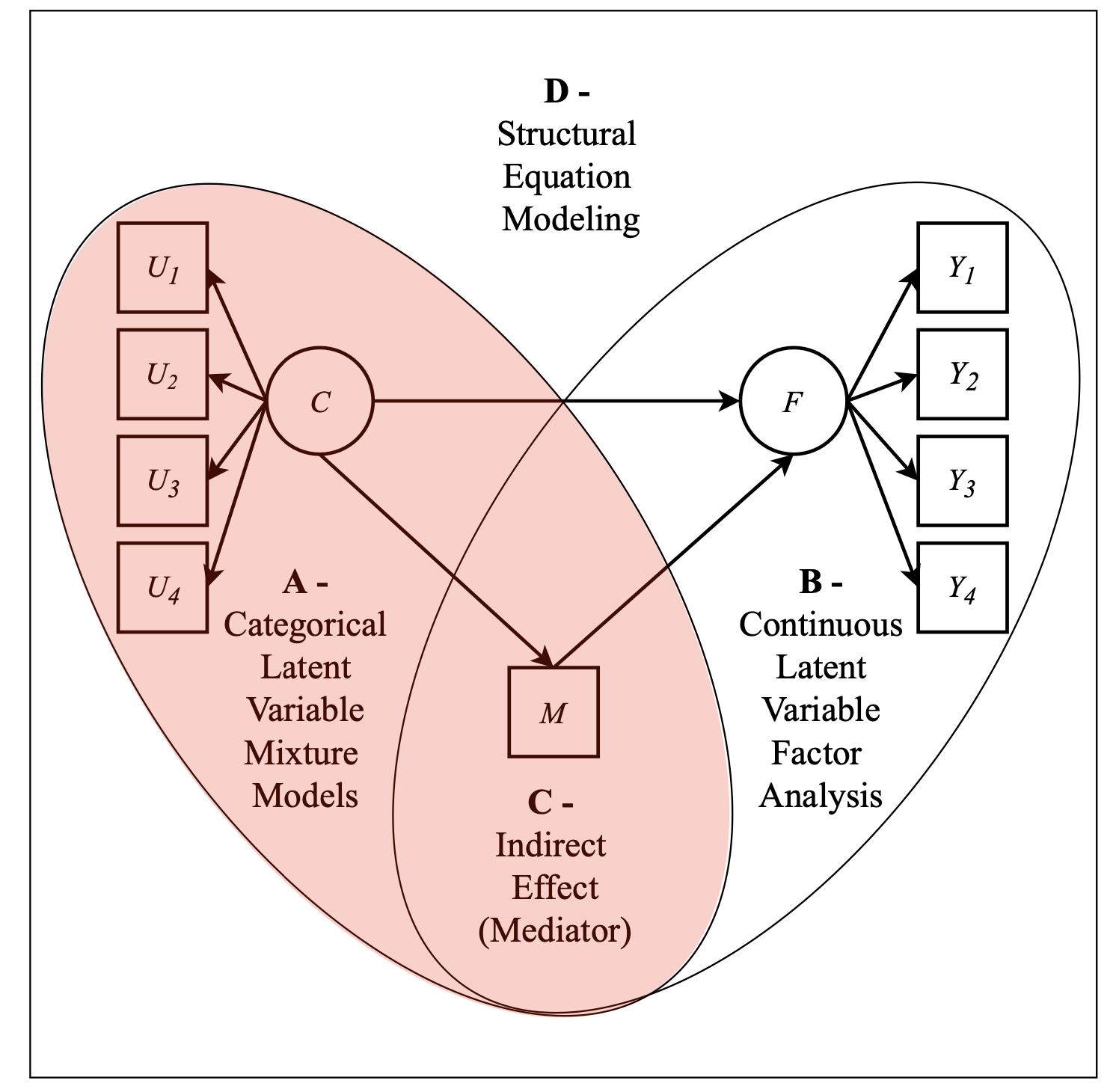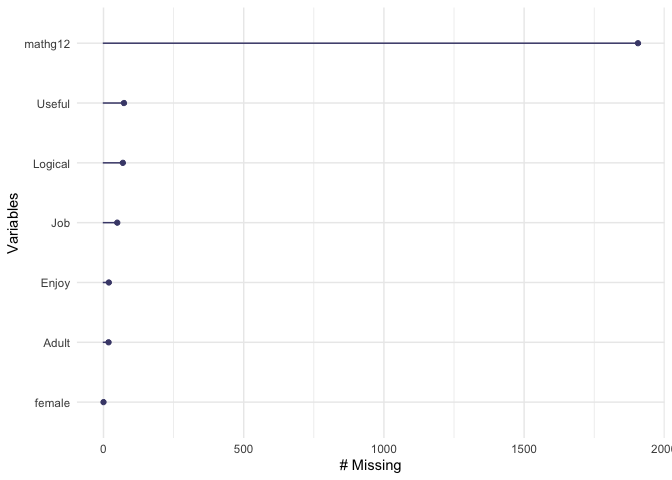# Lab 9 - The Manual 3-Step Approach - Automated

## ~~~~ Mixture Models with Covariates and Distal Outcomes ~~~~ Structural Equation Modeling - Instructor: Karen Nylund-Gibson

### May 28, 2020

University of California, Santa BarbaraFigure. Picture has been adapted from study by Múthen, 2006.

# Lab preparation

## Creating a version-controlled R-Project with Github

On the Github repository webpage:

1. fork your own branch of the lab repository
2. copy the repository web URL address from the clone or download menu

Within R-Studio:

1. click “NEW PROJECT”
2. choose option Version Control
3. choose option Git
4. paste the repository web URL path copied from the clone or download menu on Github page
5. choose location of the R-Project

## Data source:

1. The first example utilizes a dataset on undergraduate Cheating available from the poLCA package (Dayton, 1998): $$\color{blue}{\text{See documentation here}}$$

2. The second examples utilizes the public-use dataset, The Longitudinal Survey of American Youth (LSAY): $$\color{blue}{\text{See documentation here}}$$

library(naniar)
library(tidyverse)
library(haven)
library(glue)
library(MplusAutomation)
library(rhdf5)
library(here)
library(janitor)
library(gt)
library(poLCA)

Incorparating distal outcome variables with mixture models

Note: Prior to adding covariates or distals enumeration must be conducted.

$$\color{blue}{\text{See Lab 7 for examples of enumeration with MplusAutomation}}$$

# DU3step

“Dichotomous self-report responses by 319 undergraduates to four questions about cheating behavior” (poLCA, 2016).

Prepare data

data(cheating)

cheating <- cheating %>% clean_names()

df_cheat <-  cheating %>%                                  #
dplyr::select(1:4) %>%                                   #
mutate_all(funs(.-1)) %>%                                #
mutate(gpa = cheating\$gpa)

Run the DU3step model with gpa as distal outcome

m_stepdu  <- mplusObject(
TITLE = "DU3STEP add distal GPA - Lab8",
VARIABLE =
"categorical = lieexam-copyexam;
usevar = lieexam-copyexam;
auxiliary = gpa (du3step);
classes = c(2);",

ANALYSIS =
"estimator = mlr;
type = mixture;
starts = 500 100;
processors = 10;",

OUTPUT = "sampstat patterns tech11 tech14;",

PLOT =
"type = plot3;
series = lieexam-copyexam(*);",

usevariables = colnames(df_cheat),
rdata = df_cheat)

m_stepdu_fit <- mplusModeler(m_stepdu,
dataout=here("du3step_mplus", "c_lca_du3step_Lab8.dat"),
modelout=here("du3step_mplus", "c4_lca_du3step_Lab8.inp") ,
check=TRUE, run = TRUE, hashfilename = FALSE)

## Application: Longitudinal Study of American Youth, Science Attitudes

lsay_data <- read_csv(here("data", "lca_lsay_sci.csv"), na = c("9999", "9999.00")) %>%               #
clean_names() %>%                                                                                  #
dplyr::select(1:5, female, mathg12,                                                                #
Enjoy = ab39m, Useful = ab39t,                                                              #
Logical = ab39u, Job = ab39w, Adult = ab39x)                                                #

Use {naniar} to look at missing on covariates and distals

naniar::gg_miss_var(lsay_data)# Manual 3-step

Integrate covariates and distals with a mixture model ____________________________________

## Step 1

step1  <- mplusObject(
TITLE = "Step1 - 3step LSAY - Lab9",
VARIABLE =

classes = c(4);

auxiliary =   ! list all potential covariates and distals here
female        ! covariate
mathg12;      ! distal math test score in 12th grade ",

ANALYSIS =
"estimator = mlr;
type = mixture;
starts = 500 100;",

SAVEDATA =
"File=3step_savedata.dat;
Save=cprob;
Missflag= 999;",

OUTPUT = "sampstat residual tech11 tech14",

PLOT =
"type = plot3;

usevariables = colnames(lsay_data),
rdata = lsay_data)

step1_fit <- mplusModeler(step1,
dataout=here("3step_mplus", "Step1_3step_LSAY.dat"),
modelout=here("3step_mplus", "Step1_3step_LSAY.inp") ,
check=TRUE, run = TRUE, hashfilename = FALSE)

## Step 2

Extract logits for the classification probabilities for the most likely latent class

logit_cprobs <- as.data.frame(step1_fit[["results"]]
[["class_counts"]]
[["logitProbs.mostLikely"]])

Extract saved dataset which is part of the mplusObject “step1_10_fit”

savedata <- as.data.frame(step1_fit[["results"]]
[["savedata"]])

Rename the column in savedata named “C” and change to “N”

colnames(savedata)[colnames(savedata)=="C"] <- "N"

Run step 2

step2  <- mplusObject(
TITLE = "Step2 - 3step LSAY - Lab9",

VARIABLE =
"nominal=N;
USEVAR = n;
missing are all (999);
classes = c(4); ",

ANALYSIS =
"estimator = mlr;
type = mixture;
starts = 0;",

MODEL =
glue(
"%C#1%
[n#1@{logit_cprobs[1,1]}];
[n#2@{logit_cprobs[1,2]}];
[n#3@{logit_cprobs[1,3]}];

%C#2%
[n#1@{logit_cprobs[2,1]}];
[n#2@{logit_cprobs[2,2]}];
[n#3@{logit_cprobs[2,3]}];

%C#3%
[n#1@{logit_cprobs[3,1]}];
[n#2@{logit_cprobs[3,2]}];
[n#3@{logit_cprobs[3,3]}];

%C#4%
[n#1@{logit_cprobs[4,1]}];
[n#2@{logit_cprobs[4,2]}];
[n#3@{logit_cprobs[4,3]}];"),

usevariables = colnames(savedata),
rdata = savedata)

step2_fit <- mplusModeler(step2,
dataout=here("3step_mplus", "Step2_3step_LSAY.dat"),
modelout=here("3step_mplus", "Step2_3step_LSAY.inp"),
check=TRUE, run = TRUE, hashfilename = FALSE)

## Step 3

Model with 1 covariate and 1 distal outcome

step3  <- mplusObject(
TITLE = "Step3 - 3step LSAY - Lab9",

VARIABLE =
"nominal=N;
usevar = n;
missing are all (999);
classes = c(4);

usevar = female mathg12;" ,

ANALYSIS =
"estimator = mlr;
type = mixture;
starts = 0;",

MODEL =
glue(
" %OVERALL%

C on female;      ! covariate as predictor of C

%C#1%
[n#1@{logit_cprobs[1,1]}];
[n#2@{logit_cprobs[1,2]}];
[n#3@{logit_cprobs[1,3]}];

[mathg12](m1);    ! conditional distal mean
mathg12;          ! conditional distal variance (freely estimated)

%C#2%
[n#1@{logit_cprobs[2,1]}];
[n#2@{logit_cprobs[2,2]}];
[n#3@{logit_cprobs[2,3]}];

[mathg12](m2);
mathg12;

%C#3%
[n#1@{logit_cprobs[3,1]}];
[n#2@{logit_cprobs[3,2]}];
[n#3@{logit_cprobs[3,3]}];

[mathg12](m3);
mathg12;

%C#4%
[n#1@{logit_cprobs[4,1]}];
[n#2@{logit_cprobs[4,2]}];
[n#3@{logit_cprobs[4,3]}];

[mathg12](m4);
mathg12; "),

MODELCONSTRAINT =
"New (diff12 diff13 diff23
diff14 diff24 diff34);

diff12 = m1-m2;  ! test pairwise distal mean differences
diff13 = m1-m3;
diff23 = m2-m3;
diff14 = m1-m4;
diff24 = m2-m4;
diff34 = m3-m4;",

MODELTEST = "     ! omnibus test of distal means
m1=m2;
m2=m3;
m3=m4;",

usevariables = colnames(savedata),
rdata = savedata)

step3_fit <- mplusModeler(step3,
dataout=here("3step_mplus", "Step3_3step_LSAY.dat"),
modelout=here("3step_mplus", "Step3_3step_LSAY.inp"),
check=TRUE, run = TRUE, hashfilename = FALSE)

# $$C_k$$ as moderator

step3mod  <- mplusObject(
TITLE = "Step3 - 3step LSAY - Lab9",

VARIABLE =
"nominal=N;
usevar = n;
missing are all (999);
classes = c(4);

usevar = female mathg12;" ,

ANALYSIS =
"estimator = mlr;
type = mixture;
starts = 0;",

MODEL =
glue(
"!!!!!!!!!!!!!!!!!!!!!!!!!!!!!!!!!!!!!!!!!!!!!!!!!!!!!!!!!!!!!
!!!!!DISTAL = mathg12, COVARIATE = female, MODERATOR = C!!!!!
!!!!!!!!!!!!!!!!!!!!!!!!!!!!!!!!!!!!!!!!!!!!!!!!!!!!!!!!!!!!!
%OVERALL%
mathg12 on female;
mathg12;

%C#1%
[n#1@{logit_cprobs[1,1]}];
[n#2@{logit_cprobs[1,2]}];
[n#3@{logit_cprobs[1,3]}];

mathg12 on female(s1);  ! conditional slope (class 1)
[mathg12](m1);          ! conditional distal mean
mathg12;                ! conditional distal variance (freely estimated)

%C#2%
[n#1@{logit_cprobs[2,1]}];
[n#2@{logit_cprobs[2,2]}];
[n#3@{logit_cprobs[2,3]}];

mathg12 on female(s2);
[mathg12](m2);
mathg12;

%C#3%
[n#1@{logit_cprobs[3,1]}];
[n#2@{logit_cprobs[3,2]}];
[n#3@{logit_cprobs[3,3]}];

mathg12 on female(s3);
[mathg12](m3);
mathg12;

%C#4%
[n#1@{logit_cprobs[4,1]}];
[n#2@{logit_cprobs[4,2]}];
[n#3@{logit_cprobs[4,3]}];

mathg12 on female(s4);
[mathg12](m4);
mathg12; "),

MODELCONSTRAINT =
"New (slope12 slope13 slope23
slope14 slope24 slope34);

slope12 = s1-s2;  ! test pairwise slope differences
slope13 = s1-s3;
slope23 = s2-s3;
slope14 = s1-s4;
slope24 = s2-s4;
slope34 = s3-s4;",

MODELTEST = " ! can run only a single Omnibus test per model
s1=s2;
s2=s3;
s3=s4;",

usevariables = colnames(savedata),
rdata = savedata)

step3mod_fit <- mplusModeler(step3mod,
dataout=here("3step_mplus", "Step3_moderation_LSAY.dat"),
modelout=here("3step_mplus", "Step3_moderation_LSAY.inp"),
check=TRUE, run = TRUE, hashfilename = FALSE)